Ex 11.2

Chapter 11 Class 11 - Intro to Three Dimensional Geometry
Serial order wise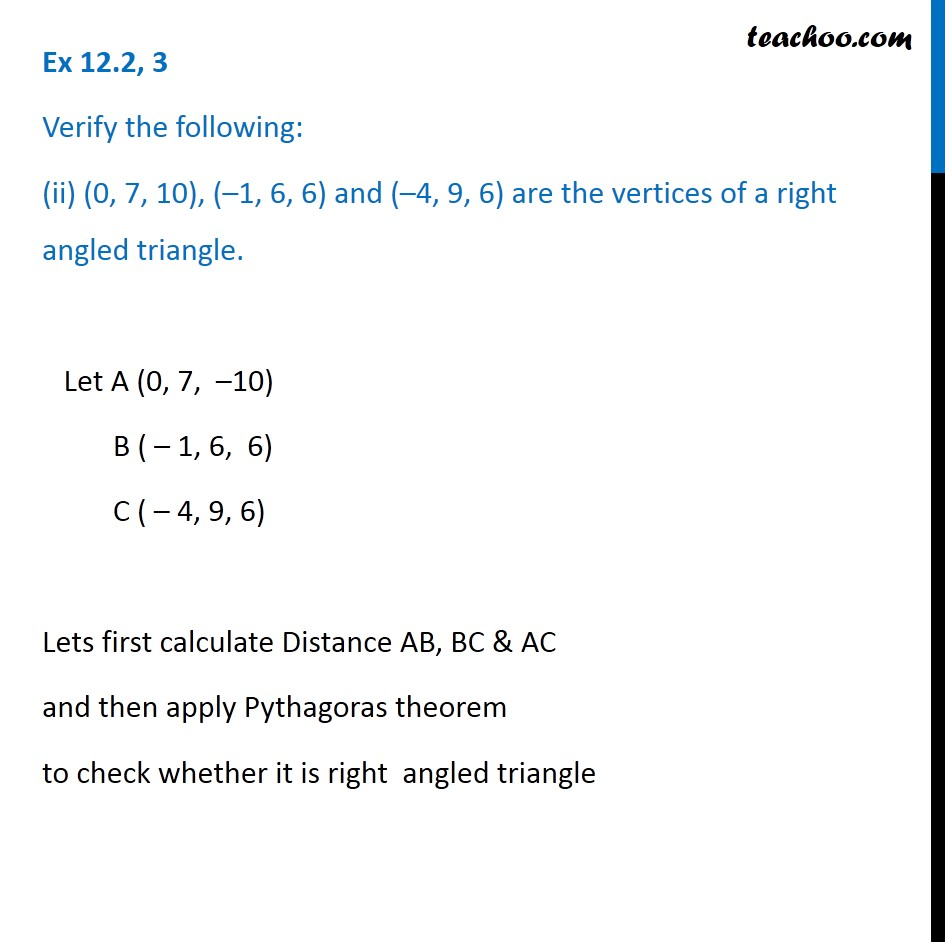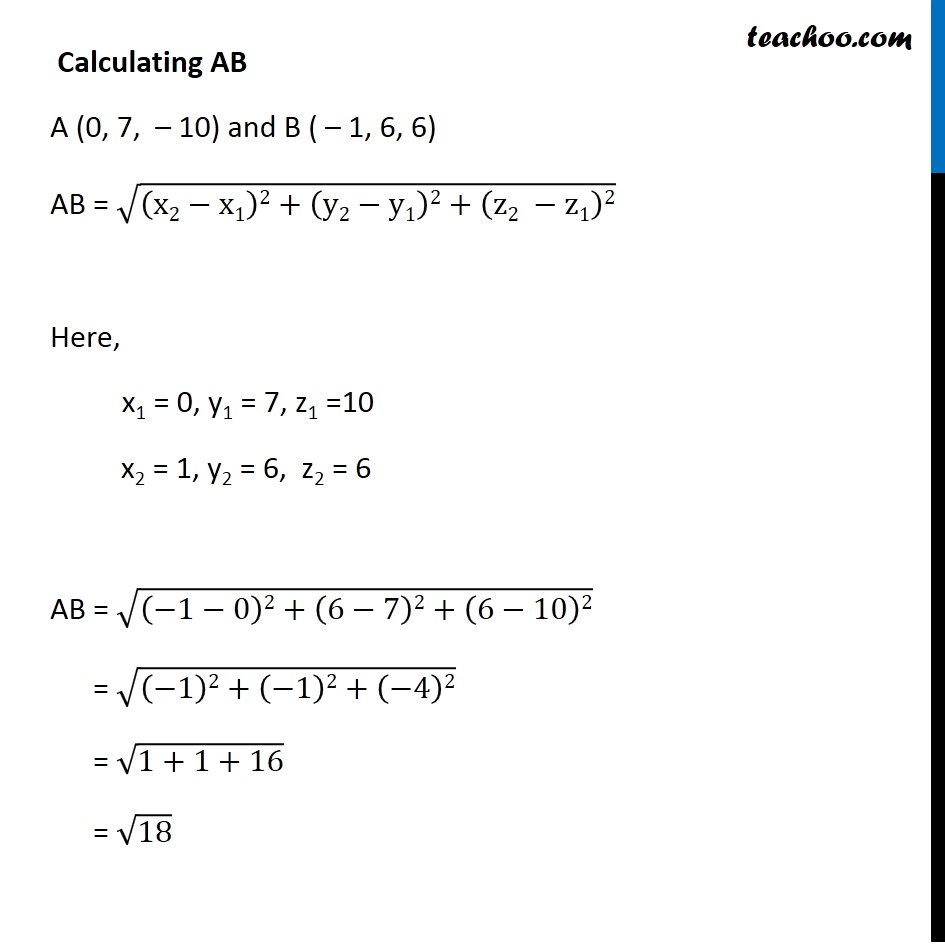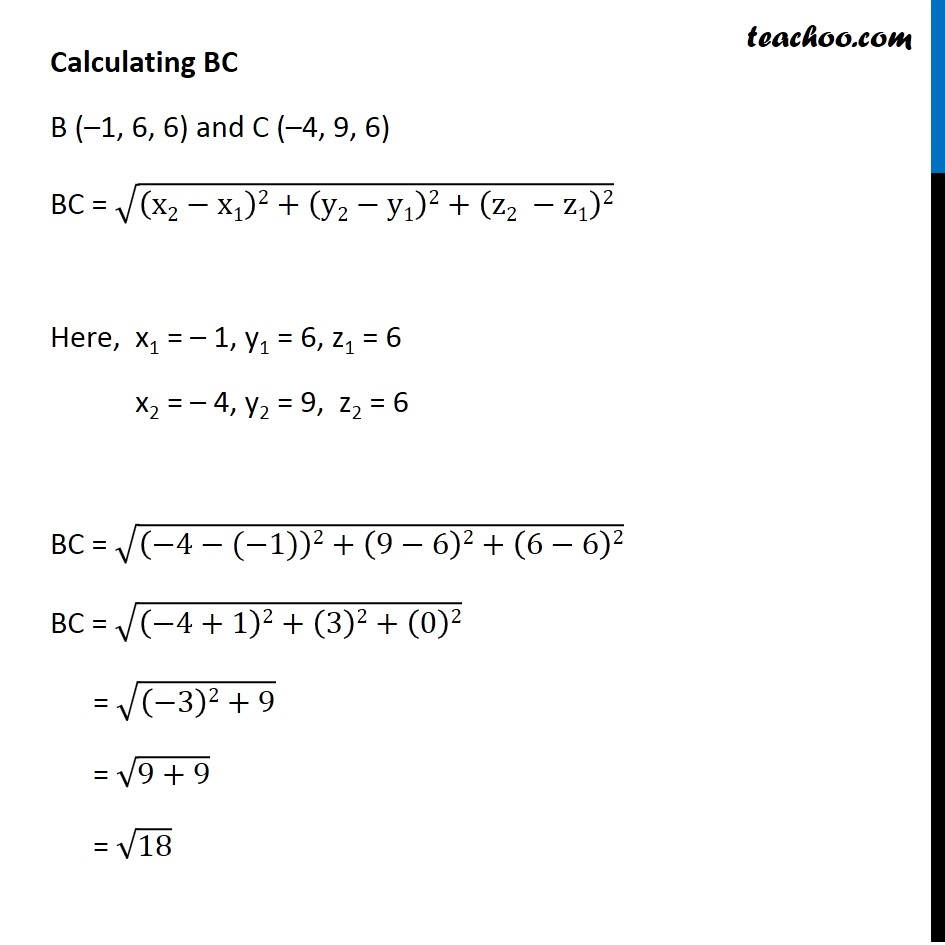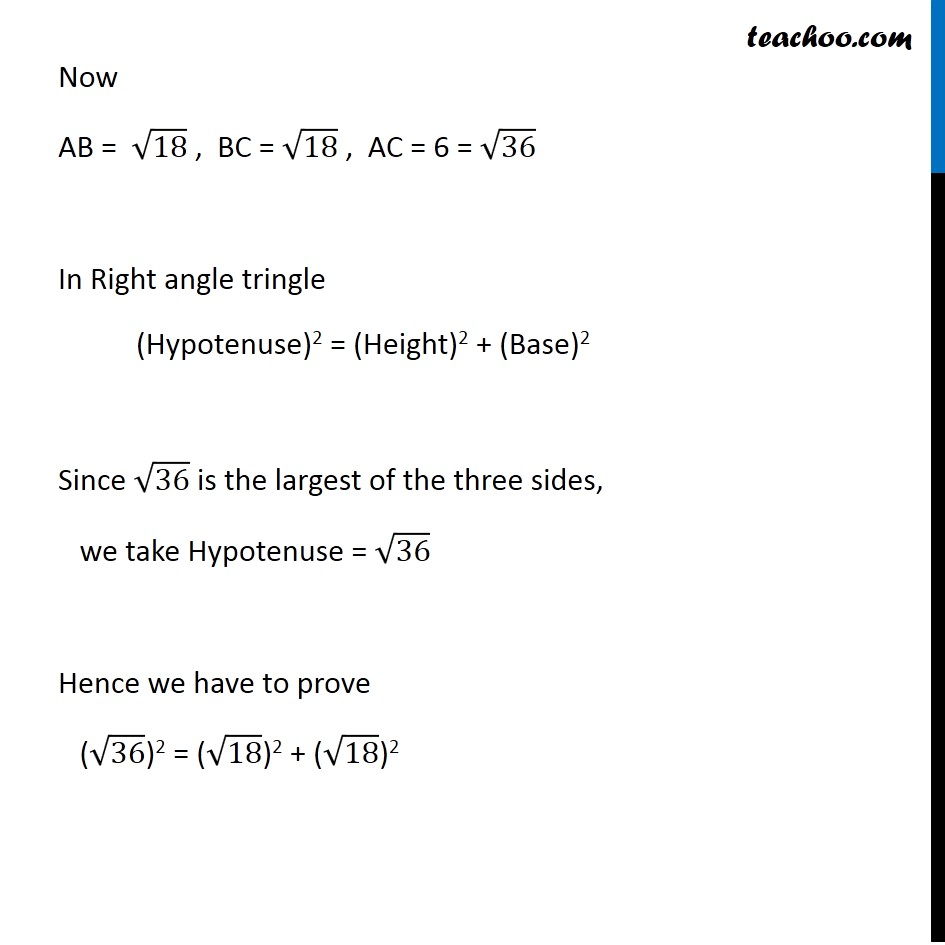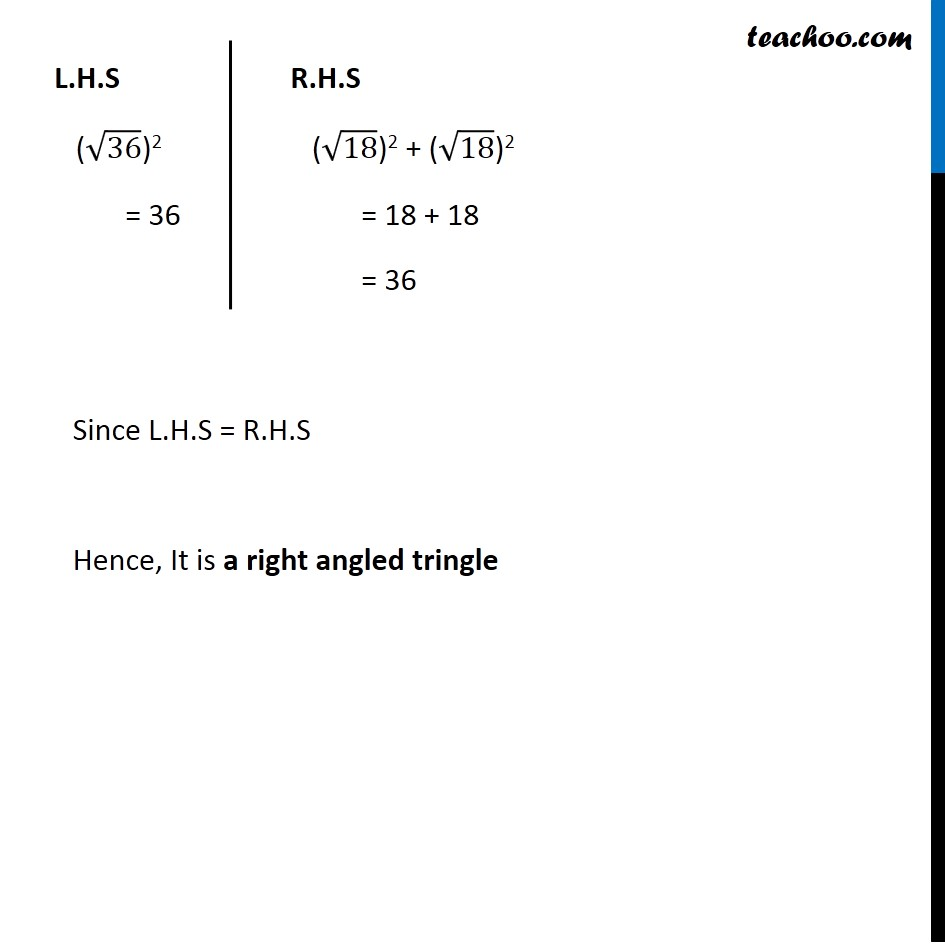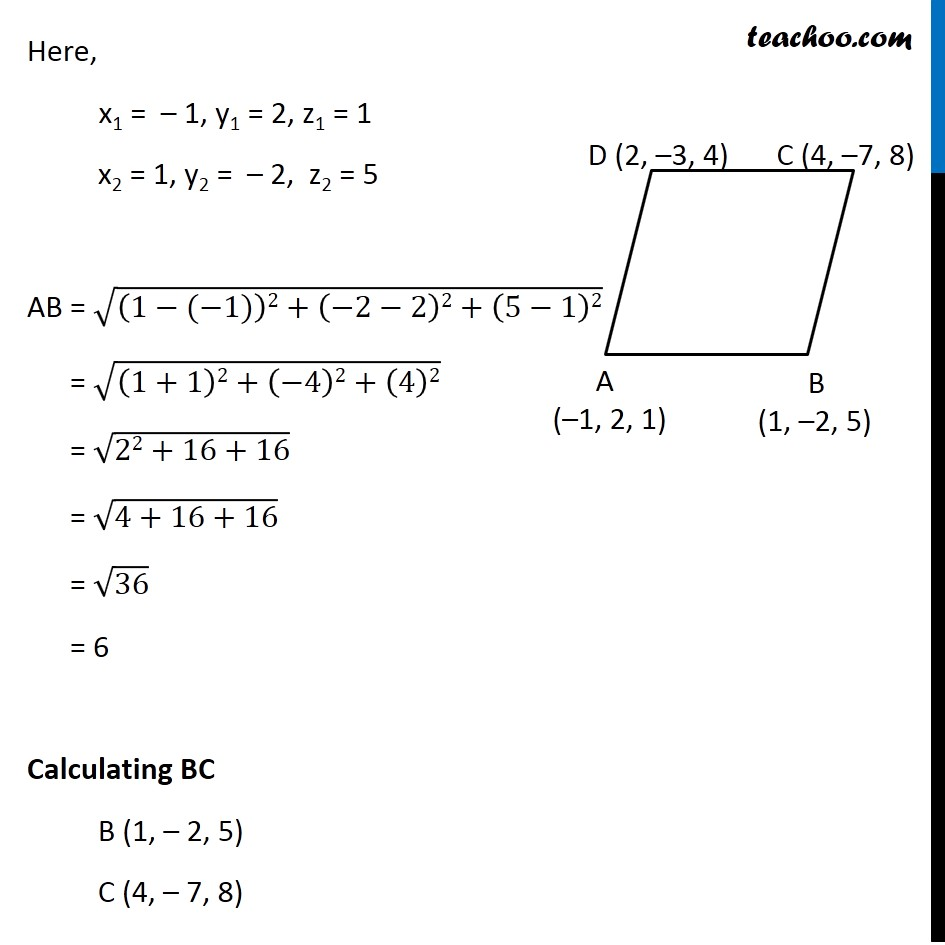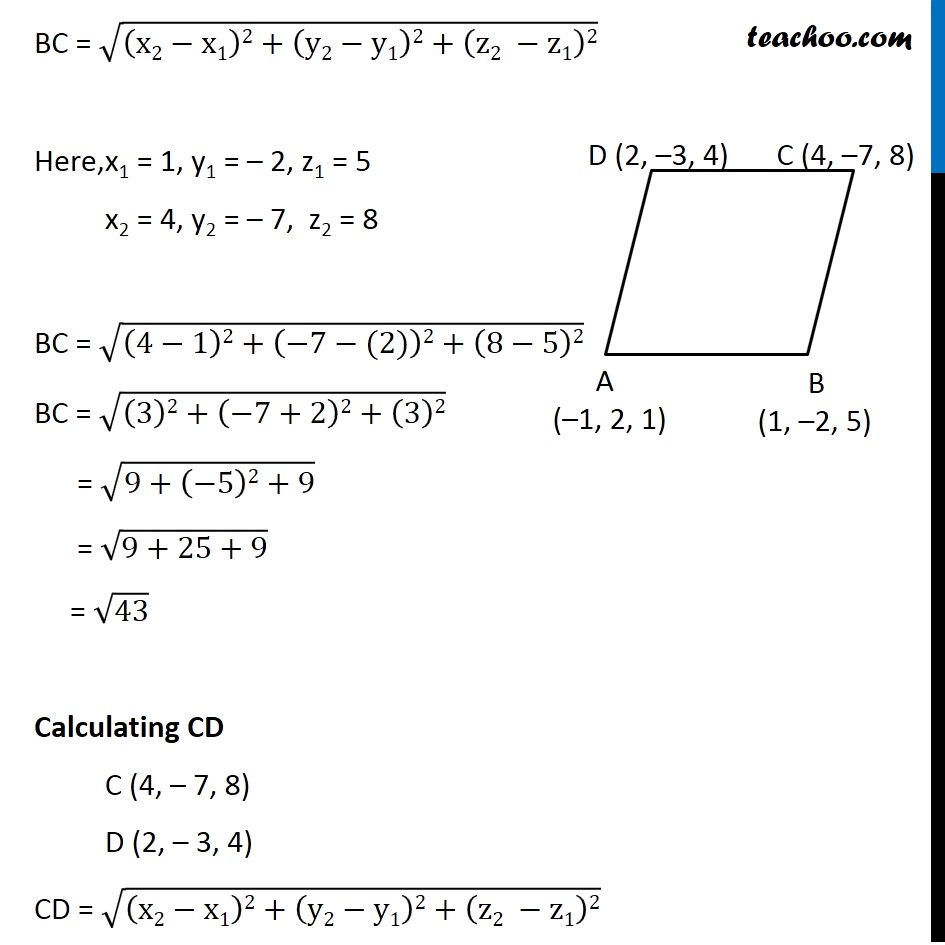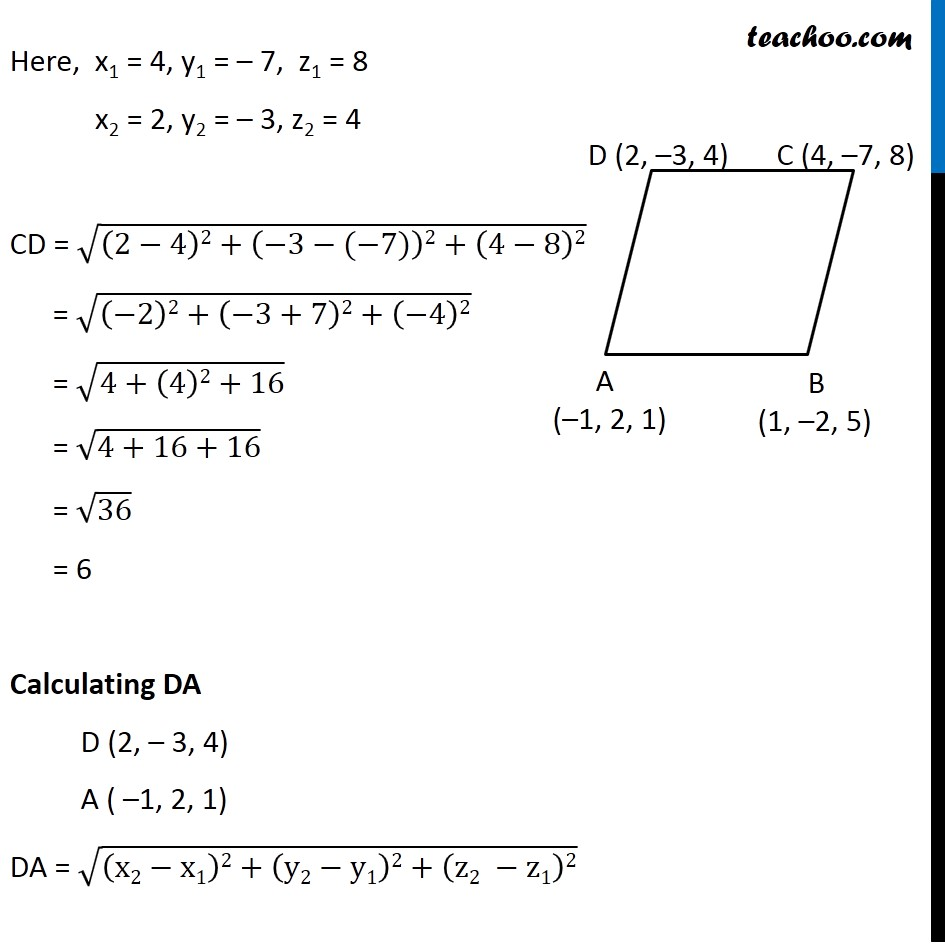Learn in your speed, with individual attention - Teachoo Maths 1-on-1 Class

### Transcript

Ex 11.2, 3 Verify the following: (ii) (0, 7, 10), (–1, 6, 6) and (–4, 9, 6) are the vertices of a right angled triangle. Let A (0, 7, –10) B ( – 1, 6, 6) C ( – 4, 9, 6) Lets first calculate Distance AB, BC & AC and then apply Pythagoras theorem to check whether it is right angled triangle Calculating AB A (0, 7, – 10) and B ( – 1, 6, 6) AB = √((x2−x1)2+(y2−y1)2+(z2 −z1)2) Here, x1 = 0, y1 = 7, z1 =10 x2 = 1, y2 = 6, z2 = 6 AB = √((−1−0)2+(6−7)2+(6−10)2) = √((−1)2+(−1)2+(−4)2) = √(1+1+16) = √18 Calculating BC B ( – 1, 6, 6) C ( – 4, 9, 6) BC = √((x2−x1)2+(y2−y1)2+(z2 −z1)2) Calculating BC B (–1, 6, 6) and C (–4, 9, 6) BC = √((x2−x1)2+(y2−y1)2+(z2 −z1)2) Here, x1 = – 1, y1 = 6, z1 = 6 x2 = – 4, y2 = 9, z2 = 6 BC = √((−4−(−1))2+(9−6)2+(6−6)2) BC = √((−4+1)2+(3)2+(0)2) = √((−3)2+9) = √(9+9) = √18 Calculating AC A (0, 7, 10) & C (–4, 9, 6) AC = √((x2−x1)2+(y2−y1)2+(z2 −z1)2) Here, x1 = 0, y1 = 7, z1 = 10 x2 = – 4, y2 = 9, z2 = 6 AC = √((−4−0)2+(9−7)2+(6−10)2) = √((−4)2+(2)2+(−4)2) = √(16+4+16) = √36 = 6 Now AB = √18 , BC = √18 , AC = 6 = √36 In Right angle tringle (Hypotenuse)2 = (Height)2 + (Base)2 Since √36 is the largest of the three sides, we take Hypotenuse = √36 Hence we have to prove (√36)2 = (√18)2 + (√18)2 L.H.S (√36)2 = 36 R.H.S (√18)2 + (√18)2 = 18 + 18 = 36 Since L.H.S = R.H.S Hence, It is a right angled tringle Ex 11.2, 3 Verify the following: (iii) (–1, 2, 1), (1, –2, 5), (4, –7, 8), and (2, –3, 4) are the vertices of a parallelogram. Let A (–1, 2, 1) , B (1, –2, 5) , C (4, –7, 8) , D (2, –3, 4) ABCD can be vertices of parallelogram only if opposite sides are equal. i.e. AB = CD & BC = AD Calculating AB A (–1, 2, 1), B (1, –2, 5) AB = √((x2−x1)2+(y2−y1)2+(z2 −z1)2) Here, x1 = – 1, y1 = 2, z1 = 1 x2 = 1, y2 = – 2, z2 = 5 AB = √((1−(−1))2+(−2−2)2+(5−1)2) = √((1+1)2+(−4)2+(4)2) = √(22+16+16) = √(4+16+16) = √36 = 6 Calculating BC B (1, – 2, 5) C (4, – 7, 8) BC = √((x2−x1)2+(y2−y1)2+(z2 −z1)2) Here, x1 = 1, y1 = – 2, z1 = 5 x2 = 4, y2 = – 7, z2 = 8 BC = √((4−1)2+(−7−(2))2+(8−5)2) BC = √((3)2+(−7+2)2+(3)2) = √(9+(−5)2+9) = √(9+25+9) = √43 Calculating CD C (4, – 7, 8) D (2, – 3, 4) CD = √((x2−x1)2+(y2−y1)2+(z2 −z1)2) Here, x1 = 4, y1 = – 7, z1 = 8 x2 = 2, y2 = – 3, z2 = 4 CD = √((2−4)2+(−3−(−7))2+(4−8)2) = √((−2)2+(−3+7)2+(−4)2) = √(4+(4)2+16) = √(4+16+16) = √36 = 6 Calculating DA D (2, – 3, 4) A ( –1, 2, 1) DA = √((x2−x1)2+(y2−y1)2+(z2 −z1)2) Here, x1 = 2, y1 = – 3, z1 = 4 x2 = –1, y2 = 2, z2 = 1 DA = √((−1−2)2+(2−(−3))2+(1−4)2) = √((−3)2+(2+3)2+(−3)2) = √(9+(5)2+9) = √(9+25+9) = √43 Since AB = CD & BC = DA So, In ABCD both pairs of opposite sides are equal Thus, ABCD is a parallelogram.